# RRB ALP 2018 Practice Test Papers | Reasoning Questions (Day-45)

Dear Aspirants, Here we have given the Important RRB ALP & Technicians Exam 2018 Practice Test Papers. Candidates those who are preparing for RRB ALP 2018 can practice these Reasoning Questions to get more confidence to Crack RRB 2018 Examination.

[WpProQuiz 2300]

Click “Start Quiz” to attend these Questions and view Solutions

1.DIRECTIONS (Qs. 1 – 2): In questions, find the odd word/letters/ number pair from the given alternatives.

(a) Kolkata

(b) Vishakhapatnam

(c) Bengaluru

(d) Haldia

1. (a) HGFE

(b) PONM

(c) DCBA

(d) MSTU

DIRECTIONS (Q. 3):

In questions below, a series is given with one term missing. Choose the correct alternative from the given ones that will complete the series.

1. 3, 6, 9, 15, 24, 39, 63,?

(a) 100

(b) 87

(c) 102

(d) 99

1. If A = l, AGE = 13, then CAR =?

(a) 19

(b) 20

(c) 21

(d) 22

1. After interchanging ÷ and =, 2 and 3 which one of the following statements becomes correct?

(a) 15 = 2 ÷ 3

(b) 5 ÷ 15 = 2

(c) 2 = 15 ÷ 3

(d) 3 = 2 ÷ 15

1. Pointing to a man, a lady said “His mother is the only daughter of my mother”. How is the lady related to the man?

(a) Mother

(b) Daughter

(c) Sister

(d) Aunt

1. After walking 10 m, Shankar turned left and covered a distance of 6 m, then turned right and covered a distance of 20 m. In the end, he was moving towards the south. From which direction did Shankar start his journey?

(a) West

(b) North

(c) South

(d) East

1. If ‘–’ stands for ‘+’, ‘+’ stands for ‘×’, ‘×’ stands for ‘–’ then which one of the following is not correct?

(a) 22 + 7 – 3 × 9 = 148

(b) 33 × 5 – 10 + 20 = 228

(c) 7 + 28 –3 × 52 = 127

(d) 44 – 9 + 6 × 11 = 87

9.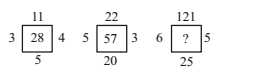(a) 176

(b) 115

(c) 157

(d) 131

1. Some equations are solved on the basis of certain system. find out the correct answer for the unsolved equation on that basis:

7 × 6 × 4 = 674,

8 × 5 × 3 = 583,

9 × 1 × 2 =?

(a) 727

(b) 292

(c) 192

(d) 462

DIRECTIONS (Qs. 1 – 2):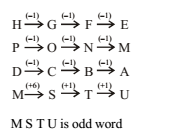DIRECTIONS (Q. 3):

3 +3 = 6

6 + 3 = 9

9 + 6 = 15

15 + 9 = 24

24 + 15 = 39

39 + 24 = 63

63 + 39 = 102

As, A + G + E = 1 + 7 + 5 = 13 Similarly, C + A + R = 3 + 1 + 18 = 22

5 = 15 ÷ 3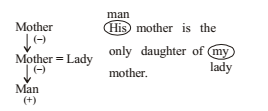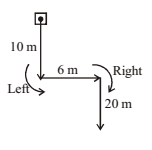By options–

(a) 22 × 7 + 3 – 9 = 148

154 + 3 – 9

157 – 9 = 148 (Correct)

(b) 33 – 5 + 10 × 20 = 228

33 – 5 + 200

200 + 33 – 5

233 – 5 = 228 (Correct)

(c)

7 × 28 + 3 – 52 = 127

196 + 3 – 52

199 – 52 = 147 (Incorrect)

(d) 44 + 9 × 6 – 11 = 87

44 + 54 – 11

98 – 11 = 87 (Correct)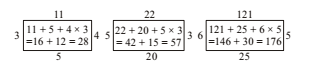As, 7 × 6 ×4 = 674,

8 × 5 × 3 = 583

Similarly, 9 × 1 × 2 = 192

RRB ALP 2018 – “All in One” Study Materials and Practice Sets